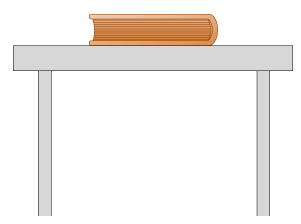# Problem: A book weighing 5 N rests on top of a table.1. A downward force of magnitude 5 N is exerted on the book by the force ofA. the tableB. gravityC. inertia 2. An upward force of magnitude _____ is exerted on the _____ by the table.A. 6N/tableB. 5N/tableC. 5N/bookD. 6N/book3. Do the downward force in Part A and the upward force in Part B constitute a 3rd law pair?A. yesB. no4. The reaction to the force in Part A is a force of magnitude_____, exerted on the _____ by the _____. Its direction is _____.A. 5N/ Earth/ Book/ UpwardB. 5N/ Book/ Table/ UpwardC. 5N/ Book/ Earth/ UpwardD. 5N/ Earth/ Book/ Downward5. The reaction to the force inPart B is a force of magnitude _____, exerted on the _____ by the_____. Its direction is _____.A. 5N/ Table/ Book/ UpwardB. 5N/ Table/ Earth/ UpwardC. 5N/ Book/ Table/ UpwardD. 5N/ Table/ Book/DownwardE. 5N/ Earth/ Book/ Downard6. Which of Newton's law dictates that the forces in Parts A and B are equal and opposite?A. Newton's 1st or 2nd lawB. Newton's 3rd law7. Which of Newton's laws dictates that the forces in Parts B and E are equal and opposite?A. Newton's 1st or 2nd lawB. Newton's 3rd law

###### FREE Expert Solution
94% (111 ratings)View Complete Written Solution
###### Problem Details

A book weighing 5 N rests on top of a table.1. A downward force of magnitude 5 N is exerted on the book by the force of

A. the table

B. gravity

C. inertia

2. An upward force of magnitude _____ is exerted on the _____ by the table.

A. 6N/table

B. 5N/table

C. 5N/book

D. 6N/book

3. Do the downward force in Part A and the upward force in Part B constitute a 3rd law pair?

A. yes

B. no

4. The reaction to the force in Part A is a force of magnitude_____, exerted on the _____ by the _____. Its direction is _____.

A. 5N/ Earth/ Book/ Upward

B. 5N/ Book/ Table/ Upward

C. 5N/ Book/ Earth/ Upward

D. 5N/ Earth/ Book/ Downward

5. The reaction to the force inPart B is a force of magnitude _____, exerted on the _____ by the_____. Its direction is _____.

A. 5N/ Table/ Book/ Upward

B. 5N/ Table/ Earth/ Upward

C. 5N/ Book/ Table/ Upward

D. 5N/ Table/ Book/Downward

E. 5N/ Earth/ Book/ Downard

6. Which of Newton's law dictates that the forces in Parts A and B are equal and opposite?

A. Newton's 1st or 2nd law

B. Newton's 3rd law

7. Which of Newton's laws dictates that the forces in Parts B and E are equal and opposite?

A. Newton's 1st or 2nd law

B. Newton's 3rd law